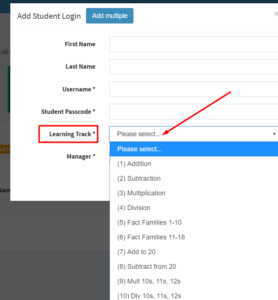## Choose a Learning Track for Online Game

### Choose from ten Learning TracksIn the Rocket Math Online Game every student needs to be started in one of the ten Learning Tracks.  A student’s Learning Track can be changed at any time**, but one must be chosen to begin with.

If you are entering the Student Login individually, you can use the pull down menu to select a learning track, as illustrated to the right.

The ten learning tracks are numbered as follows. If you are using the csv method of entry you’ll need to enter the number for the track.

2. Subtraction 1s through 9s
3. Multiplication 1s through 9s
4. Division 1s through 9s
5. Fact Familes (1 to 10) add and subtract, ex.4+5, 5+4, 9-4, 9-5
6. Fact Families (11 to 18) add and subtract, ex. 8+7, 7+8, 15+7, 15-8
7. Add to 20, example 13+4, 4+13,
8. Subtract from 20, example 15-3, 15-12,
9. Multiplication 10s-11s-12s,
10. Division 10s-11s-12s.

You can click below to see a google document showing all the problems learned in each of the Learning Tracks.

### Considerations, or what to choose when?

Begin with the basics.  The four basic operations are most important and typical expectations is one of those per grade level, so Addition in first, Addition then Subtraction in second, Multiplication, then go back to Addition and Subtraction in third, and Multiplication then Division in fourth grade, and then going back to get Addition and Subtraction if those haven’t been learned.  Make sure your student have worked through the expected basic operations for their grade level BEFORE doing any of the other optional Learning Tracks.

Another way to learn basic Addition and Subtraction Facts.  Learning in Fact Families is another order to learn. Fact Familes (1 to 10) add and subtract would be chosen in first grade.   Fact Families (11 to 18) add and subtract would be mastered in second grade.  You can choose this sequence instead of the basic addition and basic subtraction fact Learning Tracks.   Optionally, Fact Families is also a good way to review for students who have already learned the basic addition and subtraction facts in first or second grade.

Optional Learning Tracks. Add to 20 and Subtract from 20 are additional problems that the Common Core feels should be committed to memory.  They are composed of facts you can figure out if you know the basic 1s through 9s facts, but can be learned AFTER the basics are learned, if there is time in first or second grade.  They should not be assigned until after the student has mastered the basic 1s through 9s addition and subtraction facts.

After students learn the basic 1s through 9s multiplication facts, if there is time, they can move on to 10s, 11s, 12s.  After basic 1s through 9s  division facts are learned (and all the other basic operations are learned) then the 10s, 11s, and 12s are a good use of time.

**See “How to change Learning Tracks” in the FAQs and Directions document.